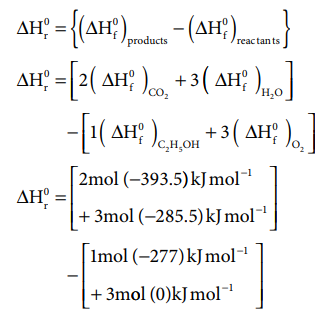Home | | Chemistry 11th std | Solved Example Problem: Thermochemical Equations

# Solved Example Problem: Thermochemical Equations

Chemistry : Thermodynamics : Thermochemical Equations

### Problem

The standard enthalpies of formation of C2H5OH(l), CO2(g) and H2O(l) are - 277, -393.5 and -285.5 kJ mol-1 respectively.

Calculate the standard enthalpy change for the reaction

C2H5OH(l)+3O2(g) ŌåÆ2CO2(g)+ 3 H2O(l)

The enthalpy of formation of O2(g) in the standard state is Zero, by definition

### Solution:

For example, the standard enthalpy change for the combustion of ethanol can be calculated from the standard enthalpies of formation of C2H5OH(l), CO2(g) and H2O(l). The enthalpies of formation are ŌĆō277, ŌĆō 393.5 and ŌĆō285.5 kJ molŌĆō1 respectively.

C3H5OH(l) + 3O2(g) ŌåÆ 2CO2(g) + 3H2O(l)=[ ŌłÆ787 ŌłÆ 856 . 5] ŌłÆ [ ŌłÆ277]

= - 1643 .5 + 277

ŌłåH0r = ŌłÆ1366 . 5 KJ

### Problem

Calculate the value of ŌłåU and ŌłåH on heating 128.0 g of oxygen from 0o C to 1000 C. CV and CP on an average are 21 and 29 J mol-1 K-1. (The difference is 8Jmol-1 K-1 which is approximately equal to R)

### Solution.

We know

ŌłåU   = n Cv (T2-T1)

ŌłåH   = n CP (T2- T1)

Here

n= 128/32 4 moles ;

T2 = 1000

C =373K;

T1 = 00

C = 273K

ŌłåU   = n Cv (T2-T1)

ŌłåU =    4 x 21 x (373 - 273)

ŌłåU =    8400 J

ŌłåU =     8.4 kJ

ŌłåH   = n Cp (T2- T1)

ŌłåH =    4 ├Ś 29 ├Ś (373- 273)

ŌłåH =    11600 J

ŌłåH =    11.6 kJ

Study Material, Lecturing Notes, Assignment, Reference, Wiki description explanation, brief detail
11th Chemistry : UNIT 7 : Thermodynamics : Solved Example Problem: Thermochemical Equations |